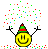# Inverse ODE, Green's Functions, and series solution

• A
• member 428835

#### member 428835

Hi PF!

One way to solve a simple eigenvalue problem like
$$y''(x)+\lambda y(x) = 0,\\ y(0)=y(1)=0$$
(I realize the solution's amplitude can be however large, but my point here is not to focus on that) is to solve the inverse problem. If we say ##A[u(x)] \equiv d^2_x u(x)## and ##B[u(x)] \equiv u(x)## then we also know that $$A^{-1}[u(x)] = \int_0^1Gu(x)\, dx,\\ B^{-1}[u(x)] = u(x)$$
where ##G## is the Green's function to the original ODE and BC.

One way to solve this problem is to let ##u(x) = \sum_{i=1}^N a_i\phi_i(x)## where ##\phi_i## is predetermined function of ##x## and ##a_i## is to be determined. This problem is know to be solved via

$$(\beta - \lambda \alpha)\textbf{ a} = \textbf{ 0},\\ \beta_{ij} =\left(B^{-1}[\phi_i],\phi_j\right) ,\\ \alpha_{ij}= \left(A^{-1}[\phi_i],\phi_j\right)$$
where ##(,)## denotes inner products and ##\textbf a## denotes the series coefficients ##a_i##. After solving this algebraic eigenvalue problem we use vector components of ##\textbf a## to approximate ##u## as a series (shown above).

My question is, since the Green's function ##G## uses the boundary conditions, is there a restriction on the selection of ##\phi_i## that requires it satisfy the boundary? In other words, can ##\phi_i = x^i## or must it be something like ##\phi_i=x^i(x-1)##?

Thanks!

No, it is not necessary that your basis functions obey the boundary conditions. Your set of basis functions must of course be "complete" in the sense that your solution can be expressed in the form ##u(x)=\sum_{i=1}^N a_i \phi_i(x)##. However, from the numerical point of view it can be advantageous, since it can accelerate the convergence.

•member 428835
eys_physics said:
No, it is not necessary that your basis functions obey the boundary conditions. Your set of basis functions must of course be "complete" in the sense that your solution can be expressed in the form ##u(x)=\sum_{i=1}^N a_i \phi_i(x)##. However, from the numerical point of view it can be advantageous, since it can accelerate the convergence.
This is what I thought, but are you sure? Attached is a plot where I have basis functions ##\phi_i = x^i##. In one case I've recombined each ##x^i## beforehand and defined ##\phi_i## to be these recombined polynomials such that they automatically satisfy the boundary conditions. Another case I simply use ##\phi_i##.

Notice recombining satisfies boundaries, but not recombining does not. Additionally, both techniques give the correct eigenvalues. It seems recombining is necessary; can you explain why not recombining won't work?

#### Attachments

• norecombine.pdf
46.6 KB · Views: 347
• recombine.pdf
51 KB · Views: 392
If you impose the boundary conditions the function ##u(x)## will satisfy them for all ##n## (i.e. the number of basis functions). On the other hand with basis functions ##\phi_i(x)=x^i##, the boundary conditions will be satisfied by your converged solution, i.e. if ##n## is large enough. Therefore, if you continue to increase ##n##, you should approach a solution satisfying the boundary conditions. Furthermore, the convergence of the coefficients in the expansion is typically slower than for the eigenvalue.

•member 428835
eys_physics said:
If you impose the boundary conditions the function ##u(x)## will satisfy them for all ##n## (i.e. the number of basis functions). On the other hand with basis functions ##\phi_i(x)=x^i##, the boundary conditions will be satisfied by your converged solution, i.e. if ##n## is large enough. Therefore, if you continue to increase ##n##, you should approach a solution satisfying the boundary conditions. Furthermore, the convergence of the coefficients in the expansion is typically slower than for the eigenvalue.
Thanks so much! I'm kind of disappointed I didn't think of trying this. That being said, do you have any literature on what you just said?

Again, thank you so much! You're a lifesaver